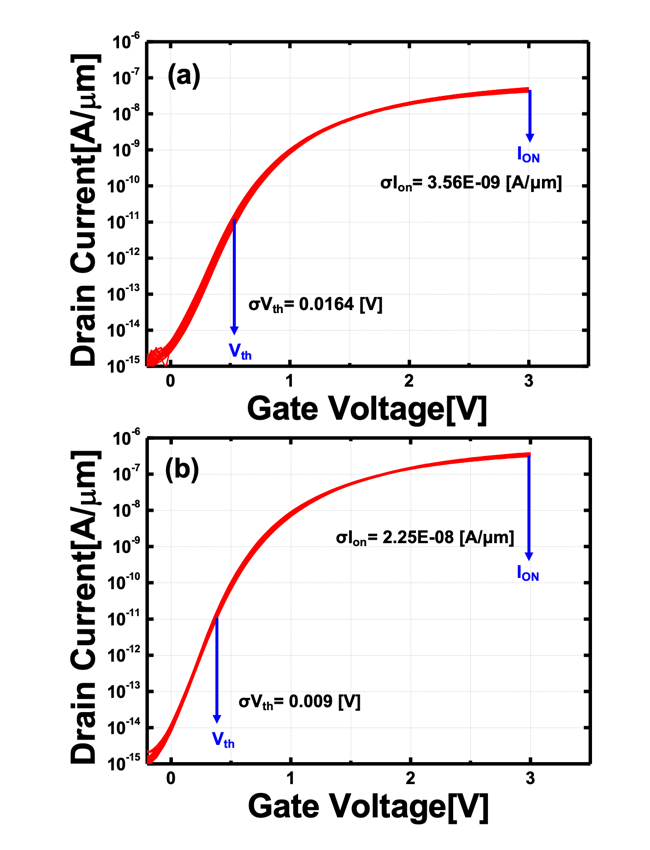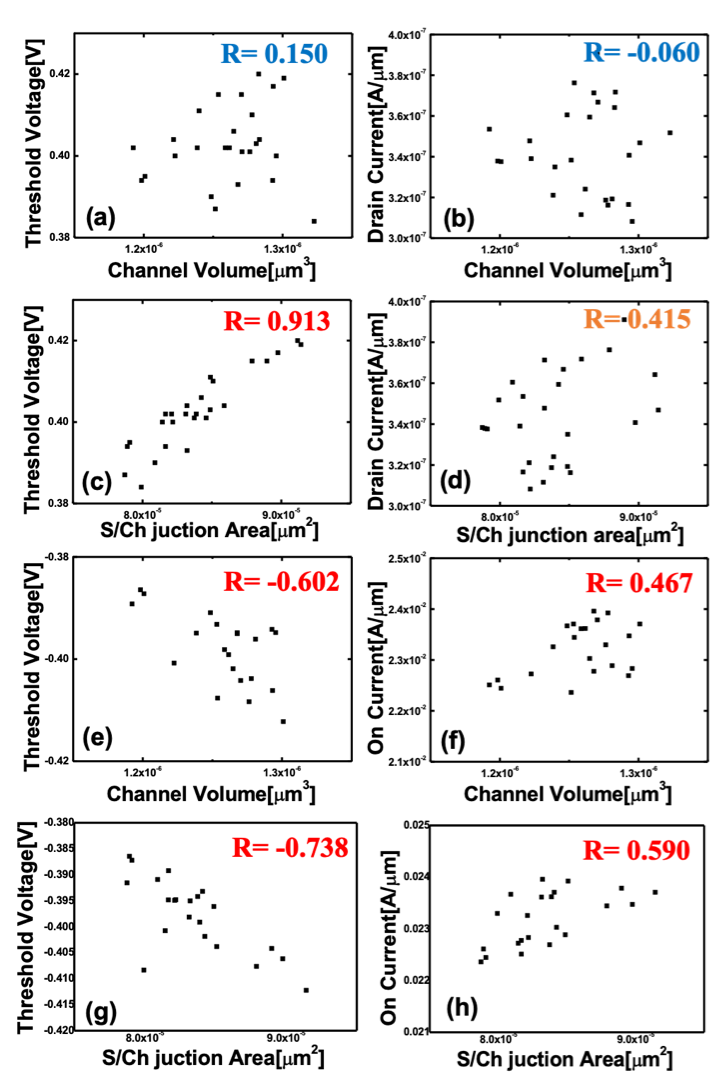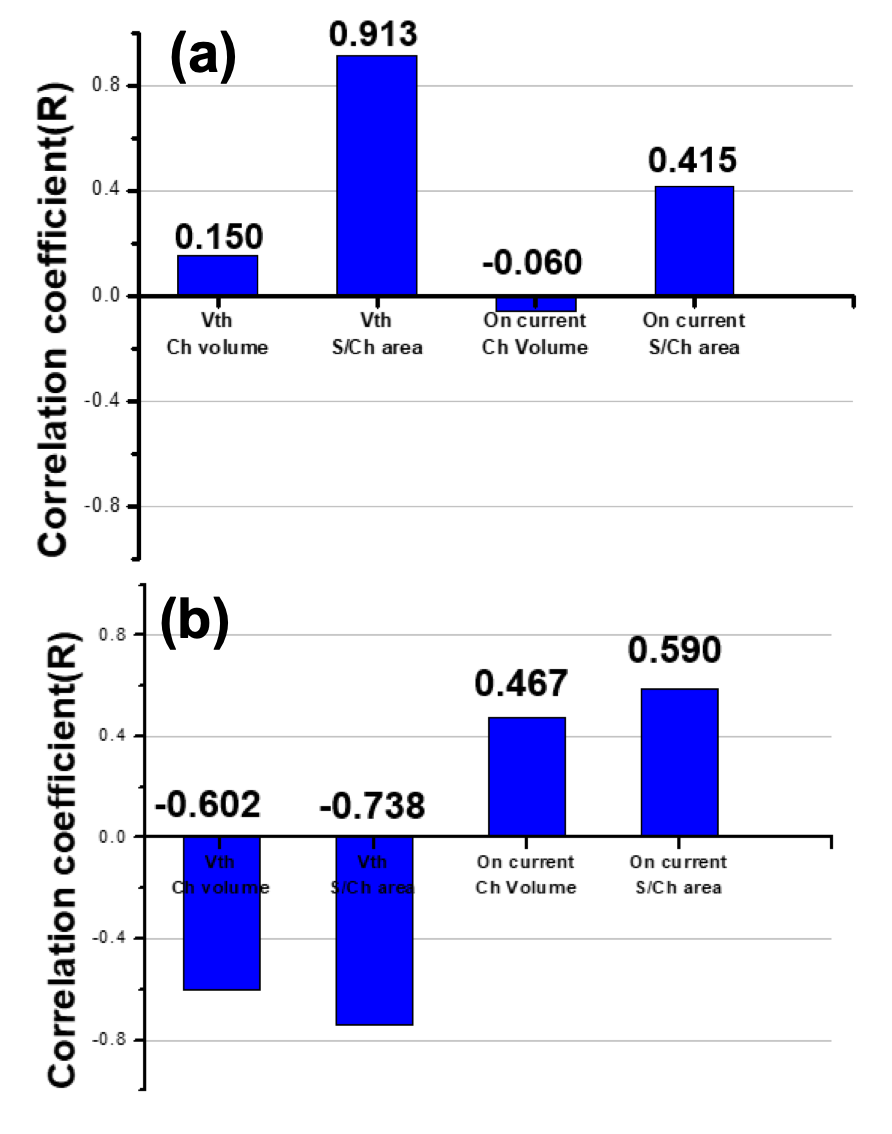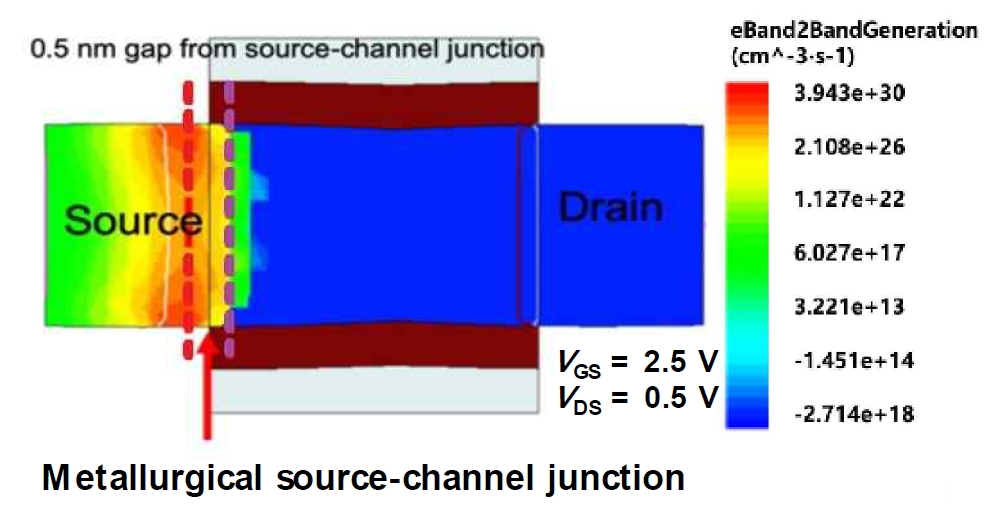Mobile QR Code1. (Inter-University Semiconductor Research Center, Department of Electrical and Computer Engineering, Seoul National University, Seoul 08826, Korea)
2. (Department of Electrical and Computer Engineering, Ajou University, Suwon 16499, Korea)

Tunnel field-effect transistor, TFET, line-edge roughness, LER effect, correlation coefficients

## I. INTRODUCTION

Recently, tunnel field-effect transistors (TFETs) have attracted many researching groups as the promising alternatives for metal-oxide-semiconductor FETs (MOSFETs) due to its low leakage current and small subthreshold swing (SS) (1-8). MOSFETs have theoretical limit of 60 mV/dec-SS at room temperature (i.e., 300 K) because their carrier injections are based on thermionic emission. Meanwhile, TFETs can overcome the limit since the function tail of Boltzmann distribution does not exist with the help of forbidden gap (1-8). In addition, the TFETs can be easily fabricated due to their good complementary MOS (CMOS) process compatibility (9-11). Therefore, there have been various attempts to demonstrate TFETs with the state-of-the-art technologies (12-19).

However, there exist various sources of process-parameter fluctuations such as random discrete dopants, oxide thickness variations and line-edge roughness (LER), which degrade the performance of FETs (16-19). Among them, LER effects are the most significant concerns with an aggressive scaling of minimum feature size (20). Previous papers have focused on changes in electrical characteristics [e.g. threshold voltages ($\textit{V}$$_{\mathrm{th}}) or ON-state current (\textit{I}$$_{\mathrm{ON}}$)] by a local dimension-variation from the LER effects (21-23). These studies suggest an easy way for an evaluation and for an insight about the effects of LER on TFETs’ electrical performances. However, there are a fundamental limit in providing quantitative analysis on how much LER effects are correlated to the electrical characteristics. Therefore, an advanced study about the LER effects in terms of the quantitative correlation factor should be performed.

This paper aims to report the influences of design parameters on the LER effects in nanowire TFETs and MOSFETs. Especially, this research focus on the channel LER since TFET’s electrical characteristics rarely depend on the channel length variation caused by gate LER (20). This paper is organized as follows. First, the structure and dimension of TFETs and MOSFETs are explained. Second, the influences of channel LER on nanowire TFETs and MOSFETs are compared comprehensively by using full three-dimensional (3D) technology computer-aided design (TCAD) simulation. Third, the channel LER effects are discussed by investigating the correlation coefficients ($\textit{R}$) for the $\textit{V}$$_{\mathrm{th}} and \textit{I}$$_{\mathrm{ON}}$ with respect to the channel volume, and source-channel junction area.

## II. SIMULATION CONDITIONS

Fig. 1. Schematic structures of (a) TFET, (b) MOSFET used in TCAD simulations. They feature nanowire channel structures with LER effects.Fig. 1 shows the structures of nanowire TFET and MOSFET to compare the channel LER effects. The 3D TCAD simulations are performed by using Synopsys Sentaurus$^{\mathrm{TM}}$ and device design parameters are summarized in Table 1 (24). Both FETs are designed to have the same physical dimension except the dopant type of source. In these structures, the LER is randomly applied to make randomized deviation to the straight channel-mask edges. The LER function is made by the power spectrum of a Gaussian autocorrelation function (24). In the Gaussian model, two parameters of root mean square (${\Delta}$) and correlation length (${\Lambda}$) have been assumed to be 2 nm and 10 nm, respectively (19). Therefore, it makes all different mask edges which have same ${\Lambda}$ and standard deviation.

Table 1. The simulation conditions for LER effect

 TFET MOSFET Channel radius ($R_{ch}$) 5 nm Channel length ($L_{ch}$) 15 nm Source/Drain length ($L_{S/D}$) 7.5 nm Oxide thickness ($t_{ox}$) 2 nm Drain doping conc. 10$^{20}$ cm$^{-3}$ (n-type) Channel doping conc. 10$^{17}$ cm$^{-3}$ (p-type) Source doping conc. 10$^{20}$ cm$^{-3}$ (p-type) 10$^{20}$ cm$^{-3}$ (n-type)

In order to rigorously examine TFET’s current-voltage characteristics, a dynamic nonlocal band-to-band tunneling (BTBT) and a Shockley-Read-Hall (SRH) generation-recombination models are used (21,22). In addition, a modified local-density approximation (MLDA) is applied to consider quantum effect. From the simulation results, $\textit{I}$$_{\mathrm{ON}} is defined as the drain current (\textit{I}$$_{\mathrm{D}}$) when gate voltage ($\textit{V}$$_{\mathrm{GS}}) is 2.5 V and drain voltage (\textit{V}$$_{\mathrm{DS}}$) is 0.5 V. The $\textit{V}$$_{\mathrm{th}} is extracted at the \textit{V}$$_{\mathrm{GS}}$ where the $\textit{I}$$_{\mathrm{D}} is 10^{4} times higher than minimum \textit{I}$$_{\mathrm{D}}$ ($\textit{I}$$_{\mathrm{OFF}}). ## III. RESULTS AND DISCUSSION Fig. 2. Transfer curves of 28 generated TFETs and MOSFETs. The \textit{V}$$_{\mathrm{th}}$ and $\textit{I}$$_{\mathrm{ON}} are extracted and indicated in each figure.Fig. 2 shows the simulated transfer curves of TFETs [Fig. 2(a)] and MOSFETs [Fig. 2(b)] under the influences of channel LER. In the simulation, 28 device structures of nanowire TFETs and MOSFETs have been generated and simulated, respectively. The SS of TFET and MOSFET are extracted to be 118 mV/dec and 151 mV/dec, respectively. The standard deviations for \textit{I}$$_{\mathrm{ON}}$ (${σ}$$\textit{I}$$_{\mathrm{ON}}$) and $\textit{V}$$_{\mathrm{th}} ({σ}$$\textit{V}$$_{\mathrm{th}}) can be extracted from the transfer curves. In (21-23), the analysis is performed for each LER case by confirming the variation of energy band diagram, {σ}$$\textit{I}$$_{\mathrm{ON}} and {σ}$$\textit{V}$$_{\mathrm{th}}. However, each analysis result cannot provide quantitative correlation for several LER cases and is valid only for cases where the analysis has been performed. In order to obtain the reliable analysis, the correlation through regression analysis should be performed. The correlation coefficient (\textit{R}) for quantity \textit{x} and \textit{y} is defined as$$\begin{equation*} R=\frac{cov\left(x,y\right)}{\sigma _{x}\sigma _{y}} \end{equation*}$$where \textit{cov}(\textit{x}, \textit{y}), {\sigma}$$_{x}$ and ${\sigma}$$_{y} are the covariance and the standard deviations for quantity \textit{x} and \textit{y}, respectively (26). The \textit{R} values are assumed to be in the range from {-}1 to +1. A +1-\textit{R} means a perfect positive correlation, while a -1-\textit{R} means a perfect negative correlation. On the other hand, a 0-\textit{R} means that there is no relationship (26). The channel LER effects are examined by extracting the \textit{R} for \textit{V}$$_{\mathrm{th}}$ and $\textit{I}$$_{\mathrm{ON}} with respect to the channel volume and source-channel junction area for the MOSFETs and TFETs, respectively (Fig. 3). Fig. 4 summarizes the \textit{R} for each case. First, in the aspect of \textit{V}$$_{\mathrm{th}}$, TFET shows the highest 0.913-$\textit{R}$ with respect to the source-channel junction area because the channel LER causes significant tunneling barrier width variation (20). On the other hand, MOSFET shows relatively high $\textit{R}$ for both the channel volume (-0.602-$\textit{R}$) and the source-channel junction area (-0.738-$\textit{R}$). It is because the $\textit{V}$$_{\mathrm{th}} of MOSFET is determined by an entire energy barrier at the channel. It is noteworthy that, unlike to the TFET, the \textit{V}$$_{\mathrm{th}}$ of MOSFET shows the negative $\textit{R}$ for both geometrical parameters; the $\textit{V}$$_{\mathrm{th}} decreases as the source-channel junction area or the channel volume increases. It is attributed to the increase of short-channel effects (SCE) (e.g., drain-induced barrier lowering) (5). On the other hand, the \textit{V}$$_{\mathrm{th}}$ of TFET is directly affected by the decrease of gate controllability as the source-channel junction area increases (i.e., positive $\textit{R}$), because it has high immunity to the SCE (8).

Fig. 3. Regression fitting curves between channel volume and (a) $\textit{V}$$_{\mathrm{th}}, (b) \textit{I}$$_{\mathrm{ON}}$ in TFETs, between source-channel junction area, (c) $\textit{V}$$_{\mathrm{th}}, (d) \textit{I}$$_{\mathrm{ON}}$ in TFETs, between channel volume, (e) $\textit{V}$$_{\mathrm{th}}, (f) \textit{I}$$_{\mathrm{ON}}$ in MOSFETs, between source-channel junction area, (g) $\textit{V}$$_{\mathrm{th}}, (h) \textit{I}$$_{\mathrm{ON}}$ in MOSFETs.Fig. 4. $\textit{R}$ value between $\textit{V}$$_{\mathrm{th}}, \textit{I}$$_{\mathrm{ON}}$, channel volume, and source-channel junction area obtained from the channel LER effect for (a) TFETs, (b) MOSFETs.Second, there is a similar correlation in terms of $\textit{I}$$_{\mathrm{ON}}. In other words, the \textit{I}$$_{\mathrm{ON}}$ of TFET mainly depends on the source-channel junction area, while the $\textit{I}$$_{\mathrm{ON}} of MOSFET depends on both the channel volume and the source-channel junction area. It is interesting that the \textit{I}$$_{\mathrm{ON}}$ of both FETs are increased although the gate controllability is decreased as the source-channel junction area or the channel volume increases; positive $\textit{R}$. It is attributed to the definition of $\textit{I}$$_{\mathrm{ON}} [Fig. 2]. It is well known that the channel surface potential is rarely modulated by \textit{V}$$_{\mathrm{GS}}$ after inversion layer formation, regardless of TFET and MOSFET (27). In short, the source-channel junction area and/or the channel volume directly determine the $\textit{I}$$_{\mathrm{ON}} (\textit{R} > 0), because the \textit{I}$$_{\mathrm{ON}}$ is extracted at high $\textit{V}$$_{\mathrm{GS}}. There is another noteworthy point in TFET that the \textit{R} for \textit{I}$$_{\mathrm{ON}}$ (0.415) is relatively lower than the $\textit{R}$ for $\textit{V}$$_{\mathrm{th}} (0.913) with respect to the source-channel junction area. Therefore, the relative weak correlation is investigated by extracting the source-channel junction area at different positions. If the area is extracted 0.5 nm away from the metallurgical source-channel junction to the source direction, \textit{R} is 0.708 (Fig. 5, red dot line). On the other hand, \textit{R} becomes 0.105 if the area is extracted 0.5 nm away from the metallurgical source-channel junction to the channel direction (Fig. 5, purple dot line). In detail, as \textit{V}$$_{\mathrm{GS}}$ increases, the source region is depleted and the location of maximum BTBT rate is shifted from the metallurgical junction to the source direction (Fig. 5). Therefore, the $\textit{V}$$_{\mathrm{th}} of TFETs shows that it depends on metallurgical junction, while \textit{I}$$_{\mathrm{ON}}$ depends on effective junction area.

Fig. 5. Cross-sectional view of nanowire-TFET. The color notes the BTBT rate.## IV. CONCLUSIONS

The influences of channel LER on the MOSFETs and TFETs have been discussed with the help of $\textit{R}$. Based on the results, we found that the $\textit{I}$$_{\mathrm{ON}} and \textit{V}$$_{\mathrm{th}}$ of the nanowire TFET are determined by the tunneling barrier width. In addition, considering the BTBT rate near source-channel junction, the region affecting $\textit{I}$$_{\mathrm{ON}} and \textit{V}$$_{\mathrm{th}}$ is very narrow. In order to control the variation in nanowire TFET's $\textit{I}$$_{\mathrm{ON}} and \textit{V}$$_{\mathrm{th}}$, more research should be performed on the tunnel junction area where $\textit{R}$ values are affected. In future works, research will have to be conducted to reduce the correlation in channel LER effect by increasing the source-channel junction.

### ACKNOWLEDGMENTS

This research was supported in part by the Brain Korea 21 Plus Project, in part by the MOTIE/KSRC under Grant 10080575 (Future Semiconductor Device Technology Development Program), in part by the NRF of Korea funded by the MSIT under Grant NRF-2019M3F3A1 A03079739 and NRF-2019M3F3A1A02072091 (Intelligent Semiconductor Technology Development Program). The EDA tool was supported by the IC Design Education Center (IDEC), Korea.

### REFERENCES

1
Sakurai T., 2004, Perspectives of low-power VLSI's, IEICE Trans. Electron., Vol. E87-C, No. 4, pp. 429-4362
Nowak E. J., 2002, Maintaining the benefits of CMOS scaling when scaling bogs down, IBM J. Res. Dev., Vol. 46, No. 2.3, pp. 169-1803
Wang P. Y., Tsui B. Y., 2016, Band engineering to improve average subthreshold swing by suppressing low electric field band-to-band tunneling with epitaxial tunnel layer tunnel FET structure, IEEE Trans. Nanotechnol., Vol. 15, No. 1, pp. 74-794
Mishra A., Pattanaik M., Sharma V., 2013, Double gate vertical tunnel FET for hybrid CMOS-TFET based low standby power logic circuits, 2013 Annual International Conference on Emerging Research Areas and 2013 International Conference on Microelectronics, Communications and Renewable Energy, pp. 1-45
Cristoloveanu S., Wan J., Zaslavsky A., 2016, A review of sharp-switching devices for ultra-low power applications, IEEE Journal of the Electron Devices Society6
Ji S., Kim H., Cho I. H., 2018, Characteristics of recess structure tunneling field effect transistor for high on current drivability, J. Semicond. Technol. Sci., Vol. 18, No. 3, pp. 360-3667
Choi W. Y., Park B. G., Lee J. D., Liu T. J. K., 2007, Tunneling field-effect transistors (TFETs) with subthreshold swing (SS) less than 60 mV/dec, IEEE Electron Device Lett., Vol. 28, No. 8, pp. 743-7458
Kim S. W., Kim J. H., Liu T. J. K., Choi W. Y., Park B. G., 2016, Demonstration of L-Shaped Tunnel Field-Effect Transistors, IEEE Trans. Electron Devices, Vol. 63, No. 4, pp. 1774-17789
Kim M., Wakabayashi Y., Nakane R., Yokoyama M., Takenaka M., Takagi S., 2014, High Ion/Ioff Ge-source ultrathin body strained-SOI tunnel FETs, 2014 IEEE International Electron Devices Meeting, pp. 13.2.1-13.2.4.10
Kim H. W., Kim J. H., Kim S. W., Sun M. C., Park E., Park B. G., 2014, Tunneling field-effect transistor with Si/SiGe material for high current drivability, Jpn. J. Appl. Phys., Vol. 53, No. 6S, pp. 06JE12-06JE12-411
Moselund K. E., Schmid H., Bessire C., Bjork M. T., Ghoneim H., Riel H., 2012, InAs-Si nanowire heterojunction tunnel FETs, IEEE Electron Device Lett., Vol. 33, No. 10, pp. 1453-145512
Tomioka K., Yoshimura M., Fukui T., 2012, Steep-slope tunnel field-effect transistors using III-V nanowire/Si heterojunction, 2012 Symposium on VLSI Technology (VLSIT), pp. 47-4813
Chen Z. X., et al. , 2009, Demonstration of tunneling FETs based on highly scalable vertical silicon nanowires, IEEE Electron Device Lett., Vol. 30, No. 7, pp. 754-75614
Conzatti F., Pala M. G., Esseni D., Bano E., Selmi L., 2012, Strain-induced performance improvements in InAs nanowire tunnel FETs, IEEE Trans. Electron Devices, Vol. 59, No. 8, pp. 2085-209215
Wang X., Brown A. R., Cheng B., Asenov A., 2011, Statistical variability and reliability in nanoscale FinFETs, 2011 International Electron Devices Meeting, pp. 5.4.1-5.4.416
Baravelli E., Dixit A., Rooyackers R., Jurczak M., Speciale N., De Meyer K., 2007, Impact of line-edge roughness on FinFET matching performance, IEEE Trans. Electron Devices, Vol. 54, No. 9, pp. 2466-247417
Patel K., Liu T. J. K., Spanos C. J., 2009, Gate line edge roughness model for estimation of FinFET performance variability, IEEE Trans. Electron Devices, Vol. 56, No. 12, pp. 3055-306318
Baravelli E., Jurczak M., Speciale N., De Meyer K., Dixit A., 2008, Impact of LER and random dopant fluctuations on FinFET matching performance, IEEE Trans. Nanotechnol., Vol. 7, No. 3, pp. 291-29819
Asenov A., Kaya S., Brown A. R., 2003, Intrinsic parameter fluctuations in decananometer MOSFETs introduced by gate line edge roughness, IEEE Trans. Electron Devices, Vol. 50, No. 5, pp. 1254-126020
Choi W. Y., 2017, Influence of line-edge roughness on multiple-gate tunnel field-effect transistors, Jpn. J. Appl. Phys., Vol. 56, No. 4S, pp. 04CD0621
Chen C., Chen Y., Fan M., Hu V. P., Su P., Chuang C., 2014, Impacts of work function variation and line-edge roughness on TFET and FinFET devices and logic circuits, 2014 SOI-3D-Subthreshold Microelectronics Technology Unified Conference (S3S), pp. 1-222
Damrongplasit N., Kim S. H., Shin C., Liu T. J. K., 2013, Impact of gate line-edge roughness (LER) versus random dopant fluctuations (RDF) on germanium-source tunnel FET performance, IEEE Trans. Nanotechnol., Vol. 12, No. 6, pp. 1061-106723
Cheng Y. H., 2019, Comparison of MOSFET threshold voltage extraction methods with temperature variation, IEEE International Conference on Microelectronic Test Structures, pp. 126-13124
Synopsys Inc. , 2009, Sentaurus Device User Guide - v.K-2015.0625
Kane E. O., Jan 1961, Theory of tunneling, J. Appl. Phys., Vol. 32, No. 1, pp. 83-9126
Terada K., Nishiyama K., Ichi Hatanaka K., 2001, Comparison of MOSFET-threshold-voltage extraction methods, Solid. State. Electron., Vol. 45, No. 1, pp. 35-4027
Kim S. W., Choi W. Y., 2016, Hump Effects of Germanium/Silicon Heterojunction Tunnel Field-Effect Transistors, IEEE Trans. Electron Devices, Vol. 63, No. 6, pp. 2583-2588## Author

Jang Hyun Kim was born in Seoul, South Korea, in 1985.

He received B.S. degree in KAIST in Daejeon in South Korea, in 2007.

He received the M.S., and Ph.D. degrees in electrical engineering from Seoul National University, Seoul, South Korea, in 2009, and 2016, respectively.

His interests include low-power CMOS device and Thin film transistor.

Seong-Su Shin was born in Seongnam, Korea, in 1992.

He received a B.S. degree at Ajou university in 2018.

He is studying for a M.S. degree in electrical and computer engineering at Ajou Univ.

His interests include tunnel fieldeffect transistor (TFET) and contact resistance.

Min-Gyu Lee was born in Suwon, Korea, in 1993.

He received a B.S. degree at Ajou university in 2019.

His interests include tunnel fieldeffect transistor (TFET).

Sangwan Kim was born in Daegu, South Korea, in 1983.

He received the B.S., M.S., and the Ph.D. degrees in Electrical Engineering from Seoul National University, Seoul, South Korea, in 2006, 2008, and 2014, respectively.

He had been a postdoctoral scholar from 2014 to 2017 at the Department of Electrical Engineering and Computer Sciences, University of California, Berkeley, USA.

Since 2017, he has been a Faculty Member with Ajou University, Suwon, South Korea, where he is currently an Assistant Professor with the Department of Electrical and Computer Engineering.